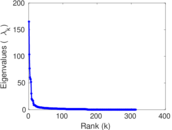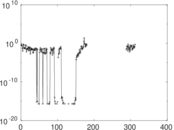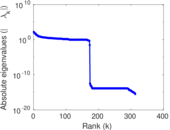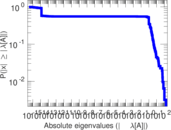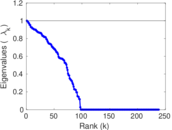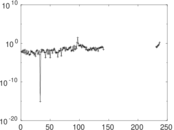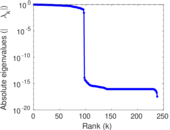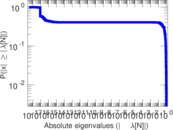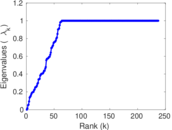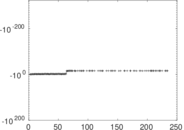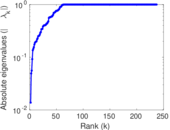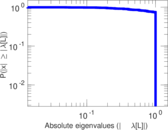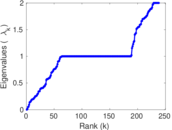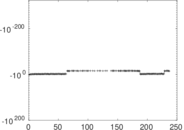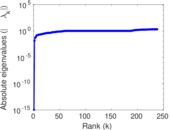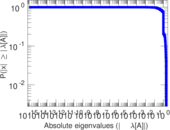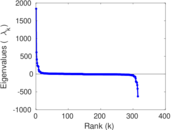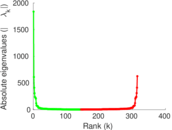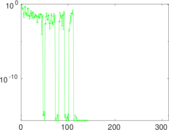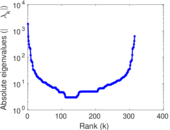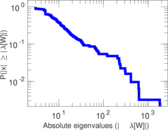# Wikiquote edits (sah)

This is the bipartite edit network of the Sakha Wikisource. It contains users and pages from the Sakha Wikisource, connected by edit events. Each edge represents an edit. The dataset includes the timestamp of each edit.

 Code `qsah` Internal name `edit-sahwikisource` Name Wikiquote edits (sah) Data source http://dumps.wikimedia.org/ AvailabilityDataset is available for download Consistency checkDataset passed all tests Category Authorship network Dataset timestamp 2017-10-20 Node meaning User, article Edge meaning Edit Network formatBipartite, undirected Edge typeUnweighted, multiple edges Temporal dataEdges are annotated with timestamps

## Statistics

 Size n = 1,832 Left size n1 = 315 Right size n2 = 1,517 Volume m = 5,882 Unique edge count m̿ = 2,663 Wedge count s = 537,345 Claw count z = 110,555,894 Cross count x = 18,338,870,281 Square count q = 116,105 4-Tour count T4 = 3,091,038 Maximum degree dmax = 2,202 Maximum left degree d1max = 2,202 Maximum right degree d2max = 188 Average degree d = 6.421 40 Average left degree d1 = 18.673 0 Average right degree d2 = 3.877 39 Fill p = 0.005 572 82 Average edge multiplicity m̃ = 2.208 79 Size of LCC N = 1,559 Diameter δ = 13 50-Percentile effective diameter δ0.5 = 2.740 08 90-Percentile effective diameter δ0.9 = 4.420 15 Median distance δM = 3 Mean distance δm = 3.271 63 Gini coefficient G = 0.758 282 Balanced inequality ratio P = 0.205 627 Left balanced inequality ratio P1 = 0.105 406 Right balanced inequality ratio P2 = 0.288 507 Relative edge distribution entropy Her = 0.759 844 Power law exponent γ = 3.337 69 Tail power law exponent γt = 1.951 00 Tail power law exponent with p γ3 = 1.951 00 p-value p = 0.186 000 Left tail power law exponent with p γ3,1 = 2.101 00 Left p-value p1 = 0.007 000 00 Right tail power law exponent with p γ3,2 = 4.541 00 Right p-value p2 = 0.000 00 Degree assortativity ρ = −0.337 879 Degree assortativity p-value pρ = 4.113 55 × 10−72 Spectral norm α = 165.061 Algebraic connectivity a = 0.013 760 2 Spectral separation |λ1[A] / λ2[A]| = 1.595 38 Controllability C = 1,416 Relative controllability Cr = 0.803 632

## Plots

### Fruchterman–Reingold graph drawing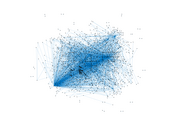### Degree distribution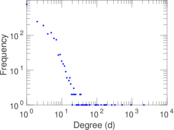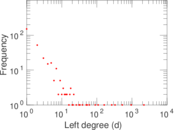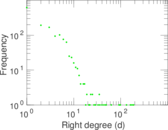### Cumulative degree distribution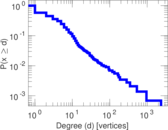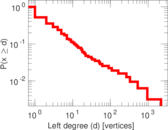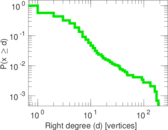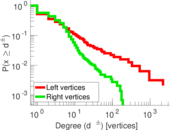### Lorenz curve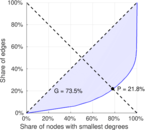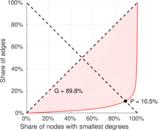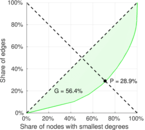### Spectral distribution of the adjacency matrix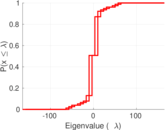### Spectral distribution of the normalized adjacency matrix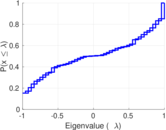### Spectral distribution of the Laplacian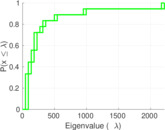### Spectral graph drawing based on the adjacency matrix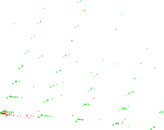### Spectral graph drawing based on the Laplacian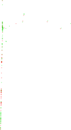### Spectral graph drawing based on the normalized adjacency matrix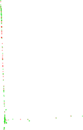### Degree assortativity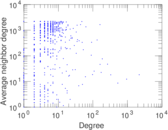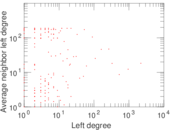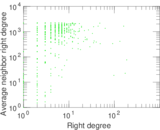### Zipf plot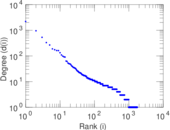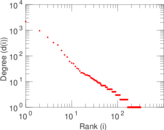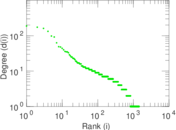### Hop distribution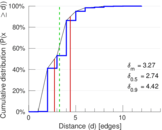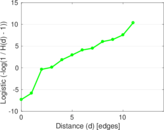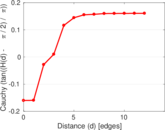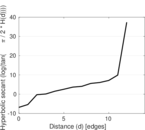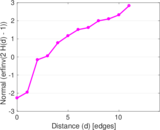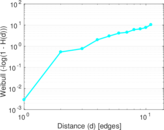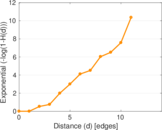### Double Laplacian graph drawing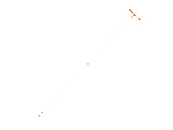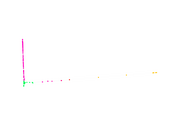### Delaunay graph drawing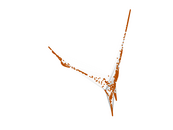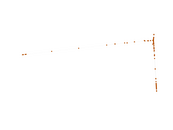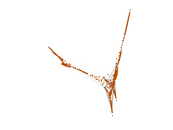### Edge weight/multiplicity distribution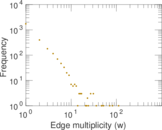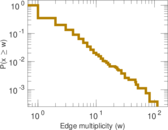### Temporal distribution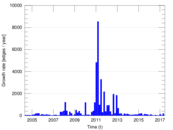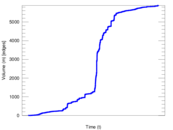### Temporal hop distribution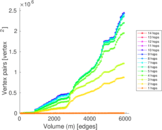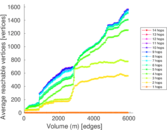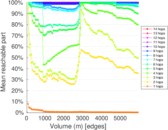### Diameter/density evolution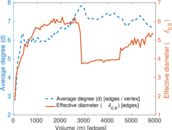### Matrix decompositions plots